Describe the matter briefly?

Difficulty: Medium

Matter exists in three states, solid, liquid, and gas. There are many properties associated with matter. For example, the matter has weight and occupies space.

There are some other propertiesthath are associated with one state of matter but not with others. For example, solids have tashape of their own while liquids and gases do not. Liquids on the other hand have definite volume while gases do not. Various materials differ in their hardness, density, solubility, flow, elasticity, conductivity, and many other qualities. The kinetic molecular model helps in understanding the properties of matter in a simplified way.

How the kinetic molecular model of matter helps differentiate various states of matter?

Difficulty: Hard

Kinetic Molecular Model of Matter:

1. The matter is made up of particles called molecules.
2. The molecules remain in continuous motion.
3. Molecules attract each other.

Characteristics of Molecular Model of Matter:

The kinetic molecular model is used to explain the three states of matter - solid, liquid, and gas.

a. Solids:

1. Solids such as stone, metal spoons, pencils, etc. have fixed shapes and volumes.
2. Their molecules are held close together by strong forces of attraction.
3. Molecules of solids vibrate about their mean positions but do not move from place to place.

b Liquids:

1. The distances between the molecules of a liquid are more than in solids. Thus, attractive forces between them are weaker.
2. Like solids, molecules of a liquid also vibrate about their mean position but are not rigidly held with each other.
3. Due to the weaker attractive forces, they can slide over one another. Thus, liquids can flow.
4. The volume of a certain amount of liquid remains the same but because it can flow hence, it attains the shape of a container in which it is put.

c. Gases:

1. Gases such as air have no fixed shape or volume. They can be filled in any container of any shape.
2. Their molecules have random motion and move with very high velocities. In gases, molecules are much farther apart than solids or liquids. Thus, gases are much lighter than solids and liquids.
3. They can be squeezed into smaller volumes.
4. The molecules of a gas are constantly striking the walls of a container. Thus, a gas exerts pressure on the walls of the container. (P ∝E)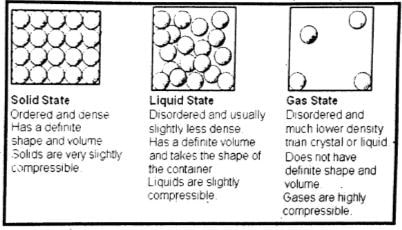d. Plasma – The fourth state of matter:

1. Production/Formation of Plasma:

The kinetic energy of gas molecules goes on increasing if a gas is heated continuously. This causes the gas molecules to move faster and faster. The collisions between atoms and molecules of the gas become so strong that they tear off the atoms. Atoms lose their electrons and become positive ions. This ionic state of matter is called plasma. Plasma is also formed in gas discharge tubes when an electric current passes through these tubes.

2. Plasma is called the fourth state of matter in which a gas occurs in its ionic state. Positive ions and electrons get separated in the presence of electric or magnetic fields.

3. Plasma also exists in neon and fluorescent tubes when they glow.

4. Most of the matter that fills the universe is in the plasma state.

5. In stars such as our Sun, gases exist in their ionic state.

6. Plasma is a highly conducting state of matter. It allows electric current to pass through it.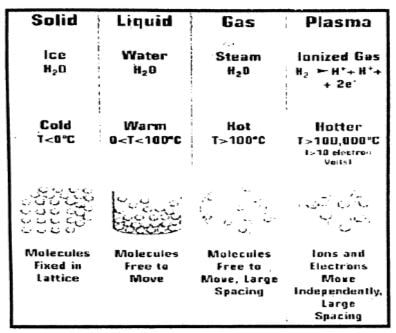##### USEFUL INFORMATION
 Density of various substance Substances Density in $kgm^{-1}$ Air 1.3 Foam 89 Petrol 800 Cooking Oil 920 Ice 920 Water 1000 Glass 2500 Aluminum 2700 Iron 7900 Copper 8900 Lead 11200 Mercury 13600 Gold 19300 Platinum 21500

##### USEFUL INFORMATION

1 metre cube (1 m3) = 1000 litre
1 litre = $10^{-3}$
1 cm3 = $10^{-6}$
1000 $kgm^{-3}$ = 1 $gcm^{-3}$

Is an iron object heavier than that of wood?

OR

Why is 1cm cubed of wood lighter than 1 cm cubed of iron?

Difficulty: Easy

Because centimeters cubed is a unit of volume, not weight. The two might take up the same amount of space, but iron is much denser and as such weighs more.

Density = $\frac{(Mass of a substance)}{(volume of the substance)}$

OR

D=$\frac{m}{v}$

What is meant by density? What is its SI unit?

Difficulty: Medium

Density:

The density of a substance is defined as its mass per unit volume.

Density =$\frac{(Mass of a substance)}{(volume of the substance)}$

OR

D=$\frac{m}{v}$

Unit of Density:

SI unit of density is kilogram per cubic meter $kgm^{-3}$).

Calculate the density of 5 liters of water?

Difficulty: Medium

Solution:

The mass of 5 liters of water is 5 kg.

Since              1 litre  =  $10^{-3} m^{3}$
5 litre  = $5 \times 10^{-3} m^{3}$
Density = $\frac{(Mass of a substance)}{(volume of the substance)}$  OR   D= $\frac{m}{v}$
Density = $\frac{(5 kg)}{(5 \times 10^{-3}} m^{3})$
= 1000 kg $m^{-3}$

The density of water is 1000 kg $m^{-3}$

Write down the density equations?

Difficulty: Medium

Density Equations:

1.     Density  = $\frac{(Mass )}{Volume}$
2.     Mass    = $Density \times Volume$
3.     Volume =$\frac{ (Mass )}{Density}$

DO YOU KNOW?

Earth's atmosphere extends upward about a few hundred kilometers with continuously decreasing density. Nearly half of its mass is between sea level and 10 km. Up to 30 km from sea level contains about 99% of the mass of the atmosphere. The air becomes thinner and thinner as we go up.

Define the term pressure?

Difficulty: Easy

Pressure:

The force acting normally per unit area on the surface of a body is called pressure.

Thus

Pressure P  = $\frac{Force}{Area}$
Or                            P = $\frac{F}{A}$

Unit of Pressure:
In SI units, the unit of pressure is $Nm^{-2}$ also called pascal (Pa).

Thus
1 $Nm^{-2}$ = 1 Pa

Show that atmosphere exerts pressure.

Difficulty: Medium

Atmospheric Pressure:

The Earth is surrounded by a cover of air called the atmosphere. It extends to a few hundred kilometers above sea level. Just as certain sea creatures live at the bottom of the ocean, we live at the bottom of a huge ocean of air. Air is a mixture of gases. The density of air in the atmosphere is not uniform. It decreases continuously as we go up. Atmospheric pressure acts in all directions.

Experiment:

Take an empty tin can with a lid. Open its cap and put some water in it. Place it over the flame. Wait till the water begins to boil and the steam expels the air out of the can. Remove it from the flame. Close the can firmly by its cap. Now place the can under tap water. The can will squeeze due to atmospheric pressure.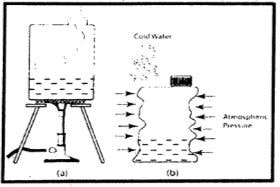When the can is cooled by tap water, the steam in it condenses. As the steam changes into water, it leaves a space behind it. This lowers the pressure inside the can as compared to the atmospheric pressure outside the can. This will cause the can to collapse from all directions. This experiment shows that the atmosphere exerts pressure in all directions.

The fact can also be demonstrated by collapsing of an empty plastic bottle when air is sucked out of it.

How soap bubbles are produced. Why the soap bubbles so formed have spherical shapes?

Difficulty: Easy

Formation of soap bubbles:

A soap bubble is a very thin sheet of water sandwiched between two layers of soap molecules. The film of soapy water surrounds a bubble of air. Soap molecules have one end that repels water, and one that attracts it, and these molecules move to the inner and outer surfaces, thrusting their water-repelling ends out in the air, and their “heads” inwards. Without such molecules on the surface, the bubble would spontaneously break apart into tiny water droplets.

The spherical shape of soap bubbles:

Surface tension causes the bubble to be spherical, which is the shape that gives the minimum surface energy – the lowest ratio of surface area to volume.

Soap bubbles expand till the pressure of air in them is equal to the atmospheric pressure.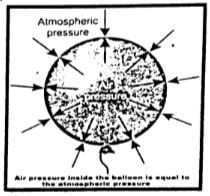Write about the factors affecting pressure?

Difficulty: Easy

Factors Affecting Pressure P  = $\frac{F}{A}$

1. The size of the force – the greater the force the greater the pressure.
2. The area of contract – the smaller the area the greater the pressure.

Write any three examples of pressure

Difficulty: Easy

Examples of Pressure:

1. Skis have a large area to hold up the weight of the skier on the snow.
2. Flat-bottomed shoes are comfortable to wear due to reduced pressure acting on our feet.
3. A sharp knife can cut easily because the very high pressure under the cutting surface is more than the object can withstand.
4. In some situations, a highly concentrated force is useful so a small area of contact is desirable e.g. scissors, knife-edge, nail point, cheese wire, spikes on golf shand oes, and the sharp edge of the spade.
5. In other situations, low pressure is better and so a large area of contact is part of the design e.g. wide tires, schoolbag straps, suitcase handles, and broad feet of elephant and camel.

What is a barometer?

OR

Write the procedure for measuring atmospheric pressure with the help of a barometer?

Difficulty: Medium

Measuring Atmospheric Pressure:

At sea level, the atmospheric pressure is about 101,300 Pa or 101,300 $Nm^{-2}$.

Barometer:

The instruments that measure atmospheric pressure are called barometers. One of the simple barometers is a mercury barometer. It consists of a glass tube 1meter long closed at one end.

Construction:

After filling it with mercury, it is inverted in a mercury trough. Mercury in the tube descends and stops at a certain height. The column of mercury held in the tube exerts pressure at its base. At sea level, the height of the mercury column above the mercury in the trough is found to be about 76 cm. The pressure exerted by 76 cm of the mercury column is nearly 101,300 $Nm^{-2}$ equals atmospheric pressure. It is common to express atmospheric pressure in terms of the height of the mercury column. As the atmospheric pressure at a place does not remain constant, hence, the height of the mercury column also varies with atmospheric pressure.DO YOU KNOW

The fan in a vacuum cleaner lowers the air pressure in its bucket. The atmospheric air rushes into it carrying dust and dirt with it through its intake port. The dust and dirt particles are blocked by the filter while the air escapes out.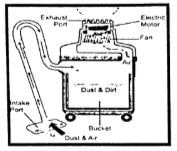Why does the atmospheric pressure vary with height?

Difficulty: Easy

Variation in atmospheric pressure:

The atmospheric pressure decreases as we go up. The atmospheric pressure on mountains is lower than at sea level. At a height of about 30 km, the atmospheric pressure becomes only 7 mm of mercury which is approximately 1000 Pa. It would become zero at an altitude where there is no air. Thus, we can determine the altitude of a place by knowing the atmospheric pressure at that place.

Atmospheric pressure may also indicate a change in the weather. On a hot day, the air above the Earth becomes hot and expands. This causes a fall in atmospheric pressure in that region. On the other hand, during cold chilly nights, the air above the Earth cools down. This causes an increase in atmospheric pressure.

What does it mean when the atmospheric pressure at a place falls suddenly?

Difficulty: Easy

The changes in atmospheric pressure at a certain place indicate the expected changes in the weather conditions of that place. For example, a gradual and average drop in atmospheric pressure means a low pressure in a neighboring locality. A minor but rapid fall in atmospheric pressure indicates a windy and showery condition in the nearby region. A decrease in atmospheric pressure is accompanied by breeze and rain. Whereas a sudden fall in atmospheric pressure is often followed by a storm, rain, and typhoon to occur in a few hours.

What change is expected in the weather if the barometer reading shows a sudden increase?

Difficulty: Easy

On the other hand, increasing atmospheric pressure with a decline, later on, predicts an intense weather condition. A gradual large increase in the atmospheric pressure indicates a long spell of pleasant weather. A rapid increase in atmospheric pressure means that it will soon be followed by a decrease in atmospheric pressure indicating poor weather ahead.

Why does atmospheric pressure change with altitude?

Difficulty: Medium

Atmospheric pressure reduce with altitude for two reasons-both related to gravity.

• The gravitation attraction (g) between the earth and air molecule is greater for those molecules nearer to earth than those further away – they have more weight – dragging them close together and increasing the pressure (force per unit area) between them.
• Molecules further away from the earth have less weight (because gravitational attraction is less) but they are also ‘standing’ on the molecules below them, causing compression. The lower down have to support more molecules above them and are further compressed (pressurized) in the process.

Note:  It is gravitational force minus the effect of the Earth’s spin (an effect that is greatest at the equator)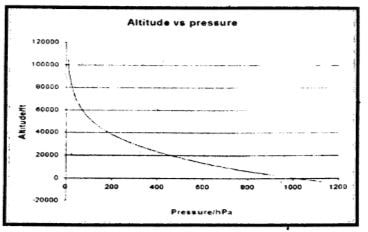DO YOU KNOW?

When air is sucked through a straw with its other end dipped in a liquid, the air pressure in the straw decreases. This causes the atmospheric pressure to push the liquid up the straw.

State relation for pressure beneath a liquid surface to depth and density?

OR

Prove that (P = rgh)

Difficulty: Medium

Pressure in Liquids:

Consider a surface of area A in a liquid at a depth of h. The length of the cylinder of liquid over this surface will be h.

The force acting on this surface will be the weight w of the liquid above this surface. If r is the density of the liquid and m is the mass of liquid above the surface, then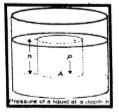Mass of the liquid cylinder = m = $volume\times density$
= $(A\times h) \times \rho$

Force acting on Area F = w = mg
= $A h \rho g$
As, Pressure P = $\frac{F}{A}$
= $\frac{(A h \rho g)}{A}$
Liquid pressure at depth h = P = $\rho g h$    ………(i)

Equation i) gives the pressure at a depth h in a liquid of density $\rho$. It shows that the pressure in a liquid increase with depth. $(P \alpha h)$

DO YOU KNOW?

The piston of the syringe is pulled out. This lowers the pressure in the cylinder. The liquid from the bottle enters the piston through the needle.State Pascal’s law.

Difficulty: Medium

Pascal’s law:

Pressure, applied at any point of a liquid enclosed in a container, is transmitted without loss to all other parts of the liquid.

Experiment:

It can be demonstrated with the help of a glass vessel having holes all over its surface.

Fill it with water. Push the piston. The water rushes out of the holes in the vessel with the same pressure. The force applied to the piston exerts pressure on the water. This pressure is transmitted equally throughout the liquid in all directions.

In general, this law holds good for fluids both liquids as well as gases.

Applications of Pascal’s law:

Pascal's law finds numerous applications in our daily life such as automobiles, hydraulic brake systems, hydraulic jacks, hydraulic presses, and other hydraulic machines.

Explain the working of a hydraulic press.

OR

A small force applied on the smaller piston results in a large force on the larger piston. Why?

Difficulty: Medium

Hydraulic Press:
A hydraulic press is a machine that works on Pascal's law. It consists of two cylinders of different cross-sectional areas. They have fitted with pistons of cross-sectional areas a and A.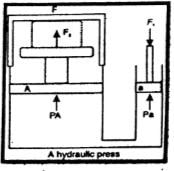The object to be compressed is placed over the piston of a large cross-sectional area A. The force $F_{1}$ is applied to the piston of the small cross-sectional area a. The pressure P produced by the small piston is transmitted equally to the large piston and a force $F_{2}$ acts on A which is much larger than $F_{1}$.
Pressure on the piston of the small area a is given by

P = $\frac{(F_{1})}{a}$
Applying Pascal's law, the pressure on the large piston of area A will be the same as on the small piston.
P  =$\frac{(F_{2})}{A}$

Comparing the above equations, we get
$\frac{(F_{1})}{a}$ = $\frac{(F_{2})}{A} F_{2}$ = $A \times \frac{(F_{1})}{a}$
Or, $F_{2}$ = $F_{1} \times\frac{(A)}{a}$  ………………..(i)

Notes: Since the ratio $\frac{(A)}{a}$ is greater than 1, hence the force $F_{2}$ that acts on the larger piston is greater than the force $F_{1}$ acting on the smaller piston. Hydraulic systems working in this way are known as force multipliers.

Explain the braking system in a vehicle?

Difficulty: Easy

The braking systems of cars, buses, etc. also work on Pascal's law. The hydraulic brakes allow equal pressure to be transmitted throughout the liquid. When the brake pedal is pushed, it exerts a force on the master cylinder, which increases the liquid pressure in it. The liquid pressure is transmitted equally through the liquid in the metal pipes to all the pistons of other cylinders. Due to the increase in liquid pressure, the pistons in the cylinders move outward pressing the brake pads with the brake drums. The force of friction between the brake pads and the brake drums stops the wheels.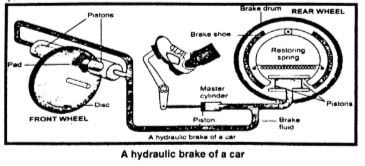State Archimedes principle.

Difficulty: Hard

Then, $h_{2} – h_{1}$ = h
If $P_{1}$ and $P_{2}$ are the liquid pressures at depths $h_{1}$ and $h_{2}$ respectively and $\rho$ is its density, then according to the equation
$P_{1}$    =    $\rho g h_{1}$
$P_{2}$     =    $\rho g h_{2}$

Let the force $F_{1}$ be exerted at the cylinder top by the liquid due to pressure $P_{1}$ and the force $F_{2}$ is exerted at the bottom of the cylinder by the liquid due to $P_{2}$.

$F_{1}$ = $P_{1}$ A    =$\rho gh_{1}A$

And, $F_{2}$ = $P_{2}$ A = $\rho g$ $h_{2}$A
$F_{1}$ and $F_{2}$ are acting on the opposite faces of the cylinder.

Therefore, the net force F will be $F_{2}$ – $F_{1}$ in the direction of $F_{2}$. This net force F on the cylinder is called the upthrust of the liquid.
$F_{2} – F_{1}$ = $\rho g A (h_{2}- h_{1})$

Or, Up thrust of liquid = $\rho g (A h)$
(Ah = V)

Or, = $\rho g V$
= $( \rho V ) g$ $( \rho V = m )$
= mg         ……………..(i)

Here Ah is the volume V of the cylinder and is equal to the volume of the liquid displaced by the cylinder. Therefore,  $\rho gV$ is the weight of the liquid displaced. Equation (i) shows that an upthrust acts on the body immersed in a liquid and is equal to the weight of the liquid displaced, which is the Archimedes principle.

DO YOU KNOW?

Hydrometer:

A hydrometer is a glass tube with a scale marked on its stem and heavyweight in the bottom. It is partially immersed in a fluid, the density of which is to be measured. One type of hydrometer is used to measure the concentration of acid in a battery. It is called acid meter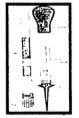Determine the density of an object using the Archimedes principle?

Difficulty: Hard
The density of an object:
Archimedes' principle is also helpful to determine the density of an object. The ratio in the weights of a body with an equal volume of liquid is the same as in their densities.

Let, the Density of the object = D
Density of the liquid  = $\rho$
Weight of the object = $w_{1}$
Weight of equal volume of liquid = w = $w_{1} – w_{2}$

Here $w_{2}$ is the weight of the solids in a liquid.  According to Archimedes' principle, $w_{2}$ is less than its actual weight $w_{1}$ by an amount w.

Since, $\frac{(D )}{\rho}$=$\frac{(w_{1})}{w}$
D = $\frac{(w_{1}- w_{2})}{w}\times\rho$

Or, D = $\frac{(w_{1})}{(w_{1}- w_{2})}\times \rho$     ………………(i)

Thus, by finding the weight of the solid in the air $w_{1}$ and its weight in water $w_{2}$, we can calculate the density of the solid by using equation (i) as illustrated in the following example.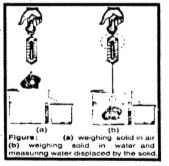What is upthrust? Explain the principle of floatation.

Difficulty: Medium

Upthrust:

Upthrust is a force that pushes an object up and makes it seem to lose weight in a fluid. (Remember, a fluid means a liquid or a gas).

The upthrust, or buoyancy, keeps ships afloat. The upthrust, or buoyancy, keeps swimmers on top of the water.

Principle of floatation:

An object sinks if its weight is greater than the upthrust acting on it. An object floats if its weight is equal to or less than the upthrust. When an object floats in a fluid, the upthrust acting on it is equal to the weight of the object.

In the case of a floating object, the object may be partially immersed. The upthrust is always equal to the weight of the fluid displaced by the object. This is the principle of floatation. It states that:

A floating object displaces a fluid having a weight equal to the weight of the object. Archimedes' principle applies to liquids as well as gases.

Explain how wooden blocks, ships, and boats move up the water surface.

Difficulty: Medium

A wooden block floats on water. It is because the weight of an equal volume of water is greater than the weight of the block. According to the principle of floatation, a body floats if it displaces water equal to the weight of the body when it is partially or completely immersed in water.

Ships and boats are designed on the same principle of floatation. They carry passengers and goods over water. It would sink in water if its weight including the weight of its passengers and goods becomes greater than the upthrust of water.

Explain how a submarine moves up the water surface down into the water.

Difficulty: Medium

Ships and submarines:

A submarine can travel over as well as underwater. It also works on the principle of floatation. It floats over water when the weight of water equal to its volume is greater than its weight. Under this condition, it is similar to a ship and remains partially above water level. It has a system of tanks that can be filled with and emptied from seawater. When these tanks are filled with seawater, the weight of the submarine increases. As soon as its weight becomes greater than the upthrust, it dives into the water and remains underwater. To come upon the surface, the tanks are emptied from seawater.

What is meant by deforming force?

Difficulty: Medium

Deforming force:

We know that the length of a rubber band increases on stretching it. Similarly, the pointer of a spring balance is lowered when a body is suspended from it. It is because the length of the spring inside the balance increases depending upon the weight of the suspended body.

The applied force that changes the shape, length, or volume of a substance is called deforming force. In most cases, the body returns to its original size and shape as soon as the deforming force is removed.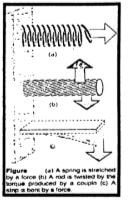What is meant by elasticity?

Difficulty: Easy

Elasticity:

The property of a body to restore its original size and shape as the deforming force ceases to act is called elasticity.

Due to elasticity, we can determine the strength of a material and the deformation under the action of a force.

Differentiate between stress and strain?

Difficulty: Medium
Stress:
The force acting on a unit area at the surface of a body is called stress.

Thus     stress    =  $\frac{Force}{Area}$
Unit:    In SI, the unit of stress is newton per square meter $(Nm^{-2})$.

Strain:
A comparison of such a change caused by the stress with the original length, volume, or shape is called strain.
If stress produces a change in the length of an object then the strain is called tensile strain.

Tensile strain    = $\frac{(Change in length)}{(Original length)}$
The strain has no units as it is simply a ratio between two similar quantities.

What is Hook’s law? What is meant by elastic limit?

Difficulty: Medium

Hook’s law:
The strain produced in a body by the stress applied to it is directly proportional to the stress within the elastic limit of the body.

Thus, $\text{stress} \propto \text{strain}$
Or, $\text{stress} = \text{constant} \times \text{strain}$
Or, $\frac{(\text{stress} )}{\text{strain}} = \text{constant}$

Elastic Limit:

The greatest stress that can be applied to a material without causing permanent deformation is called the elastic limit.

The stress point at which a material, if subjected to higher stress, will no longer return to its original shape. Brittle materials tend to break at or shortly past their elastic limit, while ductile materials deform at stress levels beyond their elastic limit.What do you know about Young’s modulus? How would you determine the young’s modulus of an object?

Difficulty: Medium
Young’s modulus:
The ratio of stress to tensile strain is called Young's modulus.

Determination of young’s modulus:
Consider a long bar of length L0 and cross-sectional area A. Let an external force F equal to the weight w stretches it such that the stretched length becomes L. According to Hooke's law, the ratio of this stress to tensile strain is constant within the elastic limit of the body.

Young’s modulus        Y      =  $\frac{Stress}{(Tensile strain)}$
Let $\triangle L$ be the change in length of the rod, then

$\triangle L$   =      L – L0
Since                           Stress      = \frac{Force}{Area}     =    \frac{F}{A}
And                             Tensile strain  =    $(L - L_{o})/( L_{o} )$   =   $\frac{(\triangle L )}{L_{o}}$
As                               Y                  = $\frac{\triangle L}{(Tensile strain)}$

= $\frac{F}{A} \times \frac{(L_{o})}{\triangle L}$
Y = $\frac{(〖FL〗_{o})}{A\triangleL}$

Unit:  SI unit of Young's modulus is newton per square metre $(Nm ^{-2})$.